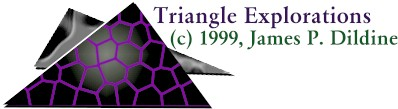James P. Dildine, 1999

Quickies:

Triangles are fascinating mathematical topics. Many other mathematical topics typically encounter or utilize elemements discovered within triangles.

Let us explore some aspects of triangles in an interactive fashion. This site will take you through some historical explorations, some interactive activities, as well as some intriguiging connections in mathematics.

A triangle in typical plane geometry is descibed as a three-sided figure or polygon whose interior angle sum is equal to 180 degrees.

The perimeter of a triangle is the sum of all three of the sides

1. Experiment with this file.

The area of a triangle is quite interesting. Often it will be represented by

Area=(1/2) x Base x Height. Where the height is an altitude drawn from the base to the opposite angle. This formula makes for a relatively easy calculation of the area of a triangle but it is rather difficult to naturally find a triangle that is given in terms of at least one side (the base) and a height. We typically can determine or are given the sides of a triangle when a triangle is present. A formula does exist that can calculate the area of a triangle when all three sides are known.

This formula is attributed to Heron of Alexandria but can be traced back to Archimedes.

This formula is represented by

Area=SQRT(s(s-a)(s-b)(s-c)),

where s=(a+b+c)/2 or perimeter/2.

This formula can be used when the height is not known and it can also be used to explore relationships between the perimeter and area of a triangle.

2. Experiment with this file.

I encourage you to experiment with the sides of triangles to explore these relationships. Use the following links to download an MS excel file that calculates the triangle area once the sides are input and also try the Java File that shows the relationship that Heron's formula has with a computer calculated area (i.e. one that we have no idea how it is calculated-a good test to see the accuracy of technology), and the typical (1/2)*Base*Height formula.

It might also be a good exercise to pose questions about the feasibility of certain triangle sides. The excel file may not give correct calculations to a certain point. Some calculations may not make sense either. This can lead to an exploration of the Triangle Inequality Theorem. You can establish the range that a third side of a triangle must fall in, if you are given two other sides and you can test this through the excel file and the Java File. Check it out and try to discover why the rules established by the Triangle Inequality Theorem would hold true.

The SUM of TWO SIDES MUST ADD UP TO BE GREATER THAN THE LENGTH OF THE REMAINING THIRD SIDE.

OR (with sides A,B,C):

• A + B > C,
• B + C> A,
• and A + C>B

So, can you have a triangle with sides 5, 12, 16?

How about 5, 12, 13? What does this look like?

Interestingly heron's formula also leads us to a result where we can calculate the height of a triangle. With base c and height h the height is,

 h = 2 (SQRT(s(s-a)(s-b)(s-c)) c

1. Imagine a triangle with a base and a vertex not on the base but on a line parallel to that base. What would happen to the perimeter and area of that triangle if the base remained the same yet the vertex was dragged all along the line parallel to the base?

Here is an activity to try...

2. Can you start a proof to heron's formula? It is rather nice to go through and very provocative. I encourage you to explore it at least in this text I have scanned.

Quickies:

Please attempt to enter various side lengths of a triangle. These Side Lengths will then be used to calcualte the area of a triangle using Heron's Formula.

Heron's Formula for Calculating the Area of a Triangle

 Sides Lengths A = B = C =
 or Area of Triangle = S = (A+B+C)/2 =>